### GATE 1998 ECE Video Solution on Digital Circuits (Digital Electronics)

1. The minimum number of 2 input NAND gates required to implement the Boolean function Z = AB’C, assuming that A, B and C are available, is
a. Two
b. Three
c. Five
d. Six

2. The noise margin of a TTL gate is about
a. 0.2 volts
b. 0.4 volts
c. 0.6 volts
d. 0.8 volts

3. In the figure shown is A = 1 and B = 1, the input B is now replaced with a sequence 101010....., the output X and Y will bea. Fixed at 0 and 1 respectively
b. X = 1010... while Y = 0101....
c. X = 1010.... and Y = 1010....
d. Fixed at 1 and 0 respectively

4. An equivalent 2’s complement representation of the 2’s complement number is 1101 is
a. 110100
b. 001101
c. 110111
d. 111101

5. An I/O processor control the flow of information between
a. Cache memory and I/O devices
b. Main memory and I/O devices
c. Two I/O devices
d. Cache and main memory

6. Two 2’s complement numbers having sign bits x and y are added and the sign bit of the result is z. Then, the occurrence of overflow is indicated by the Boolean function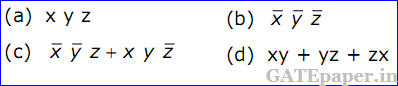7. The advantage of using a dual slope ADC in a digital voltmeter is that
a. Its conversion time is small
b. Its accuracy is high
c. It gives output in BCD format
d. It does not require a comparator

8. For the identity, AB + A’C + BC = AB + A’C, the dual form is9. An instruction used to set the carry flag in a computer can be classified as
a. Data transfer
b. Arithmetic
c. Logical
d. Program control

10. The figure is shows a mod – K counter, here K is equal to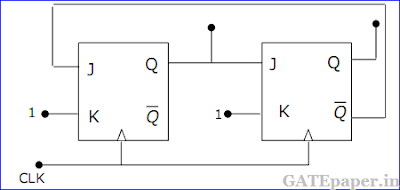a. 1
b. 2
c. 3
d. 4

11. The current I flowing through resistance ‘r’ in the circuit shown is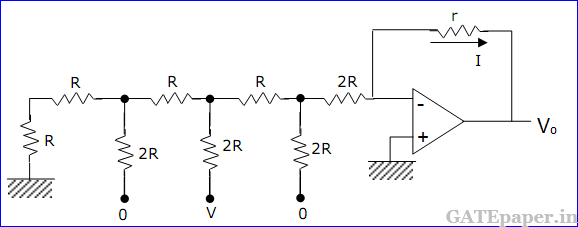a. -V/12R
b. V/12R
c. V/6R
d. V/3R

12. The K – map for a Boolean function is shown in the figure. The number of essential prime implicants for this function isa. 4
b. 5
c. 6
d. 7

13. The mod – 5 counter is shown in the figure counts through states Q2Q1Q0 = 000, 001, 010, 011 and 100.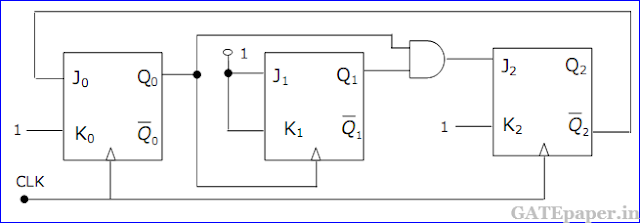a. Will the counter lockout if it happen to be in any one of the unused states?
b. Find the maximum rate at which the counter will operate satisfactorily. Assume the propagation delays of flip-flop and AND gate to be tFF and tA respectively.

14. For the TTL circuit shown in the figure, find the current flowing through the collector of transistor Q4, when Vo = 0.2 volts. Assume VCEsat = 0.2 volts, β = 100 and VBEsat = 0.7 volts. The α of Q1 in its inverse active mode is 0.01.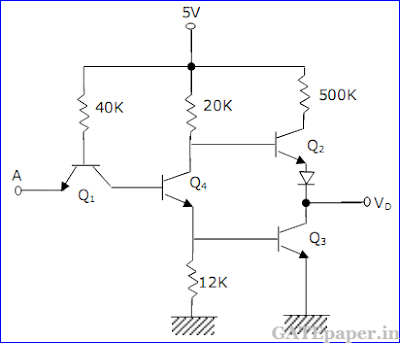15. Write a short assembly language program, without using any arithmetic instruction, to store hexadecimal 5D in the flag register of 8085 microprocessor. Data in other registers of the processor must not alter upon executing this program.

Solution :